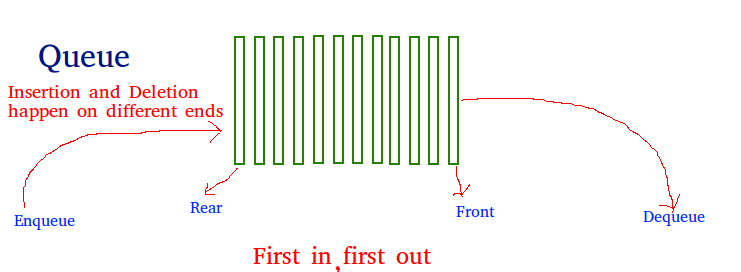Related Articles

# Queue in Python

• Difficulty Level : Easy
• Last Updated : 13 Nov, 2020

Like stack, queue is a linear data structure that stores items in First In First Out (FIFO) manner. With a queue the least recently added item is removed first. A good example of queue is any queue of consumers for a resource where the consumer that came first is served first.Operations associated with queue are:

• Enqueue: Adds an item to the queue. If the queue is full, then it is said to be an Overflow condition – Time Complexity : O(1)
• Dequeue: Removes an item from the queue. The items are popped in the same order in which they are pushed. If the queue is empty, then it is said to be an Underflow condition – Time Complexity : O(1)
• Front: Get the front item from queue – Time Complexity : O(1)
• Rear: Get the last item from queue – Time Complexity : O(1)

## Implementation

There are various ways to implement a queue in Python. This article covers the implementation of queue using data structures and modules from Python library.
Queue in Python can be implemented by the following ways:

• list
• collections.deque
• queue.Queue

#### Implementation using list

List is a Python’s built-in data structure that can be used as a queue. Instead of enqueue() and dequeue(), append() and pop() function is used. However, lists are quite slow for this purpose because inserting or deleting an element at the beginning requires shifting all of the other elements by one, requiring O(n) time.

## Python3

 `# Python program to``# demonstrate queue implementation``# using list` `# Initializing a queue``queue ``=` `[]` `# Adding elements to the queue``queue.append(``'a'``)``queue.append(``'b'``)``queue.append(``'c'``)` `print``(``"Initial queue"``)``print``(queue)` `# Removing elements from the queue``print``(``"\nElements dequeued from queue"``)``print``(queue.pop(``0``))``print``(queue.pop(``0``))``print``(queue.pop(``0``))` `print``(``"\nQueue after removing elements"``)``print``(queue)` `# Uncommenting print(queue.pop(0))``# will raise and IndexError``# as the queue is now empty`

Output:

```Initial queue
['a', 'b', 'c']

Elements dequeued from queue
a
b
c

Queue after removing elements
[]

```

```Traceback (most recent call last):
File "/home/ef51acf025182ccd69d906e58f17b6de.py", line 25, in
print(queue.pop(0))
IndexError: pop from empty list

```

#### Implementation using collections.deque

Queue in Python can be implemented using deque class from the collections module. Deque is preferred over list in the cases where we need quicker append and pop operations from both the ends of container, as deque provides an O(1) time complexity for append and pop operations as compared to list which provides O(n) time complexity. Instead of enqueue and deque, append() and popleft() functions are used.

## Python3

 `# Python program to``# demonstrate queue implementation``# using collections.dequeue`  `from` `collections ``import` `deque` `# Initializing a queue``q ``=` `deque()` `# Adding elements to a queue``q.append(``'a'``)``q.append(``'b'``)``q.append(``'c'``)` `print``(``"Initial queue"``)``print``(q)` `# Removing elements from a queue``print``(``"\nElements dequeued from the queue"``)``print``(q.popleft())``print``(q.popleft())``print``(q.popleft())` `print``(``"\nQueue after removing elements"``)``print``(q)` `# Uncommenting q.popleft()``# will raise an IndexError``# as queue is now empty`

Output:

```Initial queue
deque(['a', 'b', 'c'])

Elements dequeued from the queue
a
b
c

Queue after removing elements
deque([])

```

```Traceback (most recent call last):
File "/home/b2fa8ce438c2a9f82d6c3e5da587490f.py", line 23, in
q.popleft()
IndexError: pop from an empty deque

```

#### Implementation using queue.Queue

Queue is built-in module of Python which is used to implement a queue. queue.Queue(maxsize) initializes a variable to a maximum size of maxsize. A maxsize of zero ‘0’ means a infinite queue. This Queue follows FIFO rule.
There are various functions available in this module:

• maxsize – Number of items allowed in the queue.
• empty() – Return True if the queue is empty, False otherwise.
• full() – Return True if there are maxsize items in the queue. If the queue was initialized with maxsize=0 (the default), then full() never returns True.
• get() – Remove and return an item from the queue. If queue is empty, wait until an item is available.
• get_nowait() – Return an item if one is immediately available, else raise QueueEmpty.
• put(item) – Put an item into the queue. If the queue is full, wait until a free slot is available before adding the item.
• put_nowait(item) – Put an item into the queue without blocking. If no free slot is immediately available, raise QueueFull.
• qsize() – Return the number of items in the queue.

## Python3

 `# Python program to``# demonstrate implementation of``# queue using queue module`  `from` `queue ``import` `Queue` `# Initializing a queue``q ``=` `Queue(maxsize ``=` `3``)` `# qsize() give the maxsize``# of the Queue``print``(q.qsize())` `# Adding of element to queue``q.put(``'a'``)``q.put(``'b'``)``q.put(``'c'``)` `# Return Boolean for Full``# Queue``print``(``"\nFull: "``, q.full())` `# Removing element from queue``print``(``"\nElements dequeued from the queue"``)``print``(q.get())``print``(q.get())``print``(q.get())` `# Return Boolean for Empty``# Queue``print``(``"\nEmpty: "``, q.empty())` `q.put(``1``)``print``(``"\nEmpty: "``, q.empty())``print``(``"Full: "``, q.full())` `# This would result into Infinite``# Loop as the Queue is empty.``# print(q.get())`

Output:

```0

Full:  True

Elements dequeued from the queue
a
b
c

Empty:  True

Empty:  False
Full:  False

```

Attention geek! Strengthen your foundations with the Python Programming Foundation Course and learn the basics.

To begin with, your interview preparations Enhance your Data Structures concepts with the Python DS Course. And to begin with your Machine Learning Journey, join the Machine Learning – Basic Level Course

My Personal Notes arrow_drop_up